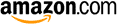Erlang C calculatorScenarios INPUT INPUT INPUT Forecast per minutequarterhalf hour INPUT INPUT INPUT Average handling time secondsminutes INPUT OUTPUT OUTPUT Number of agents Integer OUTPUT INPUT OUTPUT Average speed of answer secondsminutes OUTPUT OUTPUT INPUT Service level % waits less than secondsminutes OUTPUT OUTPUT OUTPUT Occupancy %

Examples from "Call Center Optimization" (page 4):
- FC = 100 per quarter, AHT = 3m30, number of agents = 24
- FC = 100 per quarter, AHT = 3m30, required SL = 80% within 20 seco;nds

These calculators are based on CCmath engines.
They can be implemented in any software tool and also be called from within Excel.